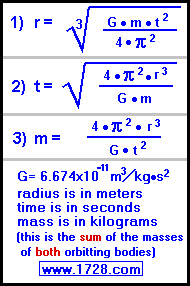Click here for a simpler Kepler's 3rd Law calculator G · m · t² = 4 · π² · r³ Kepler's 3rd Law Ultra Calculator Solves for Mass, Orbital Radius or Time Scroll to the bottom for instructions

Do you want to solve for:
Mass   Orbital Radius   or   Time ?

 M A S S *** M A S S ************** Kilograms Metric Tons Earth Mass Solar Mass Short Tons (2,000 pounds)
 Orbital Radius *** R A D I U S ********************* Meters Kilometers Miles Astronomical Units Light Years
 TIME *** T I M E *********************** seconds minutes hours days years

I N S T R U C T I O N S1) Satellites that are in geosynchronous orbit circle the Earth once per day. This eliminates the need for continuous repositioning of satellite receiving dishes because even though the satellite is moving, it stays in the same position relative to the Earth. Given that the Earth's mass is 5.9736x1024 kilograms and that the satellite's orbital period must be 86,400 seconds (one day), what altitude is required for a geosynchronous orbit?
Even though we are supposed to use the sum of the masses of both the Earth and the satellite, in this case, the satellite's mass is roughly a trillion trillion times less than the Earth and can be considered to be insignificant.
Click on the 'RADIUS' button, enter the time and mass, click on 'CALCULATE' and the answer is 4.2244 x107 meters or 42,244 kilometers or 26,249 miles. (This is the distance as measured from the Earth's center).

* * * * * * * Without Using The Calculator * * * * * * *
r3 = (G • m • t2) / (4 • π2)
r3 = (6.674x10-11 • 5.9736x1024 • 86,4002) / 39.4784
r3 = 2.976x1024 / 39.4784
r3 = 7.53922

2) The Moon orbits the Earth at a center-to-center distance of 3.86 x105 kilometers (3.86 x108 meters).
Now, look at the graphic with the formulas and you will see that the 'm' in the formula stands for the mass of both orbital bodies. Usually, the mass of one is insignificant compared to the other. However, since the Moon's mass is about ⅟81 that of the Earth's, it is important that we use the sum of their masses. Since the Moon's mass= .0735 X1024 kilograms and the Earth's mass = 5.9736 x1024 kilograms, then their sum = 6.0471 x1024 kilograms.

Now that you have this information, how long does it take the Moon to make one revolution around the Earth?

Click on the 'TIME' button. Enter the radius and mass data. Click on 'CALCULATE' and the answer is 2,371,900 seconds or 27.453 days.

* * * * * * * Without Using The Calculator * * * * * * *
t2 = (4 • π2 • r3) / (G • m)
t2 = (4 • π2 • 386,000,0003) / (6.674x10-11 • 6.0471x1024)
t2 = 2.27x1027 / 4.0414
t2 = 5,626,000,000,000
time = 2,372,000 seconds

3) Every 152,850 seconds, Io orbits Jupiter at an average orbital radius of 421,700 kilometers (4.21x108 meters). What is Jupiter's Mass?
Click on the 'MASS' button. Enter the radius and time data.
Click on 'CALCULATE' and the answer is 1.8986 x 1027 kilograms.

* * * * * * * Without Using The Calculator * * * * * * *
m = (4 • π2 • r3) / (G • t2)
m = (39.4784 • 421,700,0003) / (6.674x10-11 • 152,8502)
m = 2.961x1027 / 1.559
mass = 1.899x1027 kilograms

Significant Figures >>>
For easy readability, the answers are displayed in a "significant figure" format so you will not see answers such as 77.3333333333333333.
Numbers larger than 1,000 will be displayed in scientific notation and with the same number of significant figures specified. You may change the significant figures displayed by changing the number in the box above.
Internet Explorer and most other browsers, will display the answers properly but there are a few browsers that will show no output whatsoever. If so, enter a zero in the box above. This eliminates all formatting but it is better than seeing no output at all.